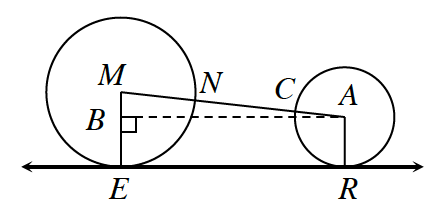### Home > GC > Chapter 10 > Lesson 10.1.5 > Problem10-48

10-48.

In the diagram at right, $⊙M$ has radius $14$ feet and $⊙A$ has radius $8$ feet. $\overleftrightarrow{ER}$ is tangent to both $⊙M$ and $⊙A$. If $NC=17$ feet, find $ER$.Think about how the radius of $⊙M$ will affect $\overline{MN}$.
How will the radius of $⊙A$ affect $\overline{AC}$?

After finding the lengths of $\overline{MN}$ and $\overline{AC}$, find lengths of the remaining sides.
$\begin{array}{l} MA=MN+NC+CA \\ ME=MN \end{array}$

$MA = 14 + 17 + 8\\ MA = 39$

$\overline{BE} ≅ \overline{AR}$ because $\overline{ME}$ and $\overline{AR}$ are perpendicular to $\overline{ER}$ by the definition of a tangent.

$ME = MB + BE\\ \;\;\; 14 = MB + 8\\ MB = 6$

Use Pythagorean Theorem to find the length of the last side.
$6^2 + (BA)^2 = 39^2\\ 36 + (BA)^2 = 1521\\ \qquad \, (BA)^2 = 1485\\ \qquad \quad \;\, BA \approx 38.535$

$BA=ER$

$ER\approx38.5\;\text{ft}$